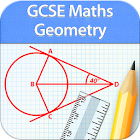GCSE Maths : Geometry Revision

All Android applications categories

All Android games categories# GCSE Maths : Geometry Revision

3 7.4

7.4 Users
rating

## Screenshots

Description

Take a breath and make your GCSE preparation a fun activity with our collection of GCSE apps. Here comes the most comprehensive Geometry app.

• HIGHEST QUALITY and QUANTITY
830 questions and 83 revision notes in all just for Geometry!. High quality content written by an experienced mathematician.

• REVISE BY TOPIC
Shapes, angles, trigonometry, measurements and scaling, transformational geometry and vectors, constructions and loci, circle theorems.

• MOCK TEST
Mixed questions from all topics.

• REVIEW with EXPLANATION
Review each question at the end of the test. Know the right answer with detailed explanation for each question.

• PROGRESS METER
With our unique progress tracking feature including pie charts and bar graphs showing your progress, you know you are ready to take on the real test at the board when your progress meter says 100%.

More details on topics:

1. Shapes - 2D and 3D:

Perimeter
Identifying 2D shapes
Polygons
Area of a rectangle
Area of a triangle
Area of a parallelogram
Area of a trapezium
Area of a compound shape
Cuboids
Prisms
Pyramids
parts of a circle
Area of a circle
Circumference of a circle
Area and sectors
Cylinders
Cones
Spheres
Compound solids

2. Angles

Angles basics
Angles in a triangle
Opposite angles
Angles around a point
Interior angles
Alternate angles
Corresponding angles
Angles and regular polygons

3. Trigonometry

Pythagoras' theorem
Pythagoras' theorem in real life
Pythagoras' theorem in 3D
Trigonometry basics
Sine
Cosine
Tangent
Sin, cos or tan?
The sine rule
The cosine rule
Using sine to fine the area of a triangle
2-D trigonometric problems
3-D trigonometric problems
Real-life problems

4. Measurements and scaling

Scales
Metric system
Imperial system
Converting measurements
Estimating measurements
Measuring time
Plans and elevations
Units of length, area and volume
Bearings
Maps and scale drawings
Nets

5. Transformational Geometry and Vectors

Congruent shapes
Lines of symmetry
Similarity
Rotational symmetry and tessellations
Planes of symmetry
Translations
reflections
Rotations
Enlargements
Enlargements 2D and 3D
Multiple transformations
Similar triangle Basics
Congruent triangles
Vectors
Vector geometry
Real life vector problems

6. Constructions and Loci

Constructing triangles
Constructing angles and perpendiculars
Loci
Real life problems

7. Circle Theorems

Angle in a semi-circle
Angle at centre is twice angle at circumference
Angles in same segment are equal
Tangents and chords
Alternate segment theorem
Circle geometry

Tags: gcse geometry quizzes

from 3 reviews

"Great"

7.4